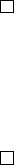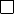Algebraic General Topology. Vol 1: Paperback / E-book || Axiomatic Theory of Formulas: Paperback / E-book

As an Amazon Associate I earn from qualifying purchases.

Pointfree binary relations
Victor Porton
November 24, 2015
Abstract
I deﬁne pointfree binary relations, a way to describe binary rela-
tions and more general structures without referring to particular “points”
(elements).
In this short article I deﬁne pointfree binary relations, that is a way
to describe binary relations and more general structures without referring to
particular “points” (elements).
Deﬁnition. Remind that binary relations (also called morphisms of the cat-
egory Rel) are triples (A, B, f) where A, B are sets and f P(A × B).
Deﬁnition. Endo-relations are relations of the form (A, A, f ) for some set A
and f P(A × A).
Deﬁnition. Isomorphism between relations µ P(A
0
×A
0
) and ν P(A
1
×
A
1
) is a bijection f : A
1
A
0
such that ν = f
1
µ f.
I remind that precategory is deﬁned as category without requirement of
existence of identity morphisms.
Deﬁnition. Ordered precategory is a precategory, each Hom-set of which
is a poset, subject to the inequalities
f
0
f
1
g
0
g
1
g
0
f
0
g
1
f
1
.
Deﬁnition. Isomorphism of ordered precategories is a map which is both
precategory isomorphism and order isomorphism.
Deﬁnition. System of pointfree relations is:
an ordered precategory (I call morphisms of which pointfree (binary)
relations; the composition is denoted );
for each Hom-sets two posets: possible domains and possible images;
domain dom and image im (maps from pointfree relations to possible
domains and possible images of its Hom-set), subject to the inequalities
f g dom f dom g im f im g.
1Deﬁnition. System of pointfree relations induced by binary relations is the
system of pointfree relations whose precategory is the category Rel of binary
relations, possible domains and images for Rel(A, B) are P A and P B corre-
spondingly and domain and image are domain and image as usually deﬁned for
binary relations.
The pointfree relation induced by a binary relation is this binary relation
considered as a pointfree binary relation.
Deﬁnition. Pointfree endo-relations are endo-relations whose source and
destination (in our precategory) is the same.
Obvious 1. System of pointfree relations induced by binary relations is really
a system of pointfree relations.
Remark. The category of pointfree relations induced by binary relations is a
large category (its set of objects is a proper class).
Deﬁnition. The isomorphism between pointfree endo-relations µ and ν of
a system of pointfree relations is an isomorphism f of the precategory of this
system such that ν = f
1
µ f.
Obvious 2. The precategory for the system of pointfree relations induced by
binary relations is a category.
Let describe reversal f 7→ f
1
of a binary relation f in pointfree terms:
f is a join (i.e., supremum) of atomic binary relation. Reversal of an atomic
relation t is deﬁned as the unique relation t
1
conforming to the formulas
dom t
1
= im t, im t
1
= dom t. So f
1
is the supremum of such t
1
. Trivially
combining these steps we get reversal of a binary relation described in pointfree
terms.
Thus we have proved:
Proposition 1. Reversal of binary relations can be restored, knowing only in-
duced pointfree relations (up to isomorphism of systems of pointfree relations).
Proposition 2. Identity relation for binary relations can be restored, know-
ing only induced pointfree relations (up to isomorphism of systems of pointfree
relations).
Proof. Identity relation id is the identity element of our semigroup (and thus
does not depend on the isomorphism).
Proposition 3. The set of bijections for relations Rel(A, B) can be restored,
knowing only induced pointfree relations (up to isomorphism of systems of point-
free relations).
Proof. Bijections f : A B are characterized by the formulas f f
1
= id
B
and f
1
f = id
A
.
2The following theorem states that pointfree endo-relations induced by bi-
nary endo-relations are essentially the same as these binary endo-relations them-
selves. Thus pointfree binary endo-relations are a generalization of binary endo-
relations.
Theorem 1. Having speciﬁed up to isomorphism (of systems of pointfree re-
lations) the system of pointfree relations induced by binary relations, we can
restore the endo-relation to which corresponds a given pointfree endo-relation
up to isomorphism (of binary relations).
Proof. Fix a set A.
Let µ Rel(A, A) be a binary relation (which we are going to restore up to
isomorphism from the corresponding pointfree relation).
We will consider possible domains and possible images D = PA.
Let A
0
be the set of atoms of D, that is one-element sets.
Take (using axiom of choice) an arbitrary bijection f : A
0
A. (Note that
it can be done using only the system of pointfree relations induced by the binary
relations up to isomorphism.)
Let y
0
, y
1
A
0
be atoms. Take elements p, q Rel(A
0
, A
0
) such that im p =
y
0
, dom q = y
1
(they exist because our system of pointfree relations is induced
by binary relations).
Deﬁne binary relation µ
0
Rel(A
0
, A
0
) as: y
0
µ
0
y
1
iﬀ q f
1
µ f p 6=
(for all y
0
, y
1
A
0
).
Thus we have restored relation µ
0
from the corresponding pointfree relation.
µ
0
was deﬁned using only properties of orders and the precategory. Thus it is
invariant under isomorphism (of systems of pointfree relations).
Let’s prove that µ
0
= f
1
µ f (and thus µ
0
is isomorphic to µ). Really,
y
0
µ
0
y
1
q f
1
µ f p 6=
x im p, y dom q : (x, y) f
1
µ f
(im p × dom q) f
1
µ f 6= y
0
(f
1
µ f ) y
1
.
If we take another isomorphism f
2
instead of f, then the induced binary
relation
µ
0
2
= f
1
2
µ f
2
= f
1
2
f µ
0
f
1
f
2
= (f
1
2
f) µ
0
(f
1
2
f)
1
.
Thus µ
0
2
is isomorphic to µ
0
(and therefore isomorphic to µ), because the com-
position f
1
2
f is a bijection. We have determined µ
0
up to isomorphism of
binary relations.
If µ
2
is a binary relation isomorphic to µ
0
, then µ
2
is isomorphic to µ and
thus µ
2
= f
1
µ f for some bijection f , so obviously µ
2
induces the same
(up to isomorphism) pointfree binary relation as µ. So we have determined
the sought-for class of pairwise isomorphic binary relations which induce (up
to isomorphism) the same pointfree binary relation as induced by µ as these
binary endo-relations which are isomorphic to µ
0
.
3
Now to the topic of both rationale and applications of this idea: I seriously
research () what I call reloids, that is ﬁlters on binary cartesian products of
sets. This seems promising to generalize for ﬁlters on pointfree relations instead
of that special case of ﬁlters on binary relations. This research is both the source
of the idea and an application of systems of pointfree relations.
Remark. Funcoids and reloids (see ) also are systems of pointfree relations.
References
 Victor Porton. Algebraic General Topology. Volume 1. 2015.
4